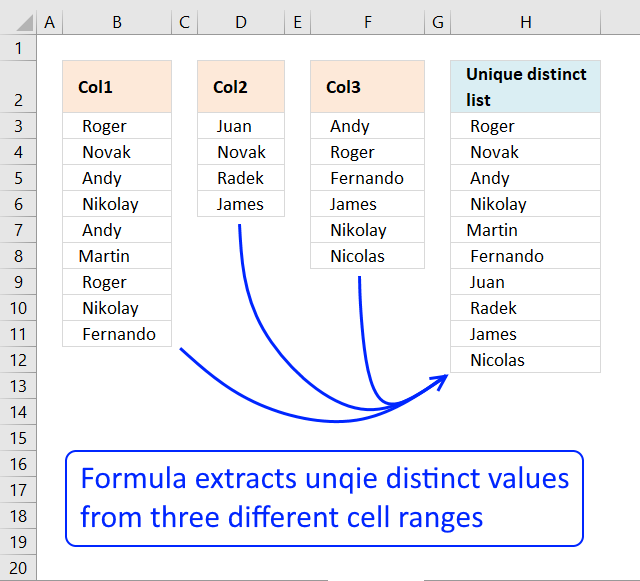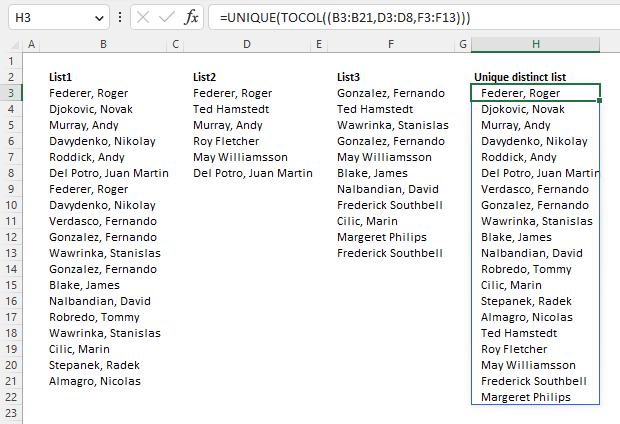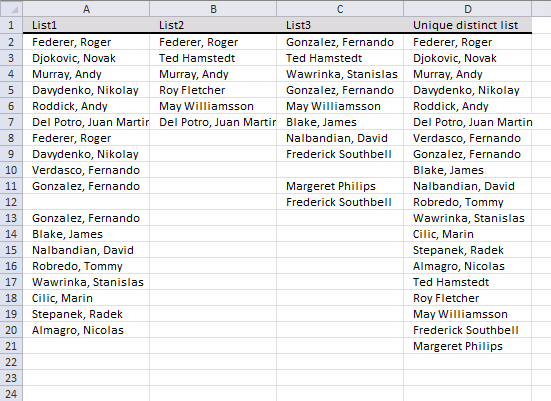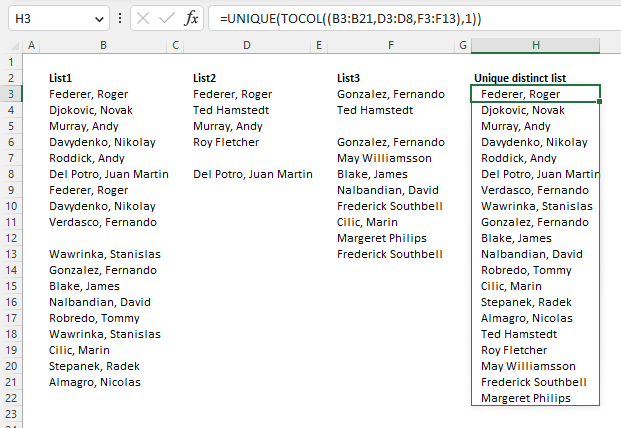Author: Oscar Cronquist Article last updated on November 23, 2022Question: How do I extract a unique distinct list from three ranges or lists? The ranges are not necessarily adjacent or the exact same length.

## 1. Extract a unique distinct list from three columnsThis formula consists of three similar parts, one returns values from Col1, the second one from col2 and the third from Col3.

IFERROR(IFERROR(formula1, formula2), formula3)

Formula in H3:

=IFERROR(IFERROR(LOOKUP(2, 1/(COUNTIF(\$H\$2:H2,\$B\$3:\$B\$11)=0), \$B\$3:\$B\$11), LOOKUP(2, 1/(COUNTIF(\$H\$2:H2, \$D\$3:\$D\$6)=0), \$D\$3:\$D\$6)),LOOKUP(2, 1/(COUNTIF(\$H\$2:H2, \$F\$3:\$F\$8)=0), \$F\$3:\$F\$8))

### 1.1 Explaining formula in cell H3

#### Step 1 - Prevent duplicate values

The COUNTIF function counts values based on a condition, in this case, I am counting values in the cells above. This makes sure that duplicates are ignored.

COUNTIF(\$H\$2:H2,\$B\$3:\$B\$11)=0

becomes

COUNTIF("Unique distinct list",{" Roger ";" Novak ";" Andy ";" Nikolay ";" Andy ";"Martin ";" Roger ";" Nikolay ";" Fernando "})=0

becomes

{0;0;0;0;0;0;0;0;0}=0

and returns

{TRUE;TRUE; TRUE;TRUE; TRUE;TRUE; TRUE;TRUE; TRUE}

#### Step 2 - Divide 1 with array

The LOOKUP function ignores errors and if we divide 1 by 0 an error occurs. 1/0 = #DIV/0!

1/(COUNTIF(\$F\$2:F2,\$B\$3:\$B\$11)=0)

becomes

1/{TRUE;TRUE; TRUE;TRUE; TRUE;TRUE; TRUE;TRUE; TRUE}

and returns

{1;1;1;1;1;1;1;1;1}

#### Step 3 - Return value based on the array

LOOKUP(2, 1/(COUNTIF(\$F\$2:F2,\$B\$3:\$B\$11)=0), \$B\$3:\$B\$11)

becomes

LOOKUP(2, {1;1;1;1;1;1;1;1;1}, \$B\$3:\$B\$21)

becomes

LOOKUP(2, {1;1;1;1;1;1;1;1;1}, {" Roger ";" Novak ";" Andy ";" Nikolay ";" Andy ";"Martin ";" Roger ";" Nikolay ";" Fernando "})

and returns " Fernando " in cell F3.

#### Step 4 - Return values from Col2

When values run out from Col1 formula1 returns errors, the IFERROR function then moves to formula2.

IFERROR(IFERROR(formula1, formula2), formula3)

formula2 is just like formula1 except that it returns values from Col2 etc.

## 2. Extract a unique distinct list from three columns - Excel 365The image above demonstrates an Excel 365 formula that extracts unique distinct values from three cell ranges combined.

Excel 365 dynamic array formula in cell H3:

=UNIQUE(TOCOL((B3:B21,D3:D8,F3:F13)))

### 2.1 Explaining formula

#### Step 1 - Merge cells

Some Excel functions allow you to combine multiple cell ranges in one argument, the TOCOL function is one of those functions.

(B3:B21,D3:D8,F3:F13)

#### Step 2 - Rearrange values

The TOCOL function rearranges values in 2D cell ranges to a single column.

Function syntax: TOCOL(array, [ignore], [scan_by_col])

TOCOL((B3:B21,D3:D8,F3:F13))

returns

{"Federer, Roger "; "Djokovic, Novak "; "Murray, Andy "; "Davydenko, Nikolay "; "Roddick, Andy "; "Del Potro, Juan Martin "; "Federer, Roger "; "Davydenko, Nikolay "; "Verdasco, Fernando "; "Gonzalez, Fernando "; "Wawrinka, Stanislas "; "Gonzalez, Fernando "; "Blake, James "; "Nalbandian, David "; "Robredo, Tommy "; "Wawrinka, Stanislas "; "Cilic, Marin "; "Stepanek, Radek "; "Almagro, Nicolas "; "Federer, Roger "; "Ted Hamstedt"; "Murray, Andy "; "Roy Fletcher"; "May Williamsson"; "Del Potro, Juan Martin "; "Gonzalez, Fernando "; "Ted Hamstedt"; "Wawrinka, Stanislas "; "Gonzalez, Fernando "; "May Williamsson"; "Blake, James "; "Nalbandian, David "; "Frederick Southbell"; "Cilic, Marin "; "Margeret Philips"; "Frederick Southbell"}

#### Step 3 - List unique distinct values

The UNIQUE function returns a unique or unique distinct list.

Function syntax: UNIQUE(array,[by_col],[exactly_once])

UNIQUE(TOCOL((B3:B21,D3:D8,F3:F13)))

becomes

UNIQUE({"Federer, Roger "; "Djokovic, Novak "; "Murray, Andy "; "Davydenko, Nikolay "; "Roddick, Andy "; "Del Potro, Juan Martin "; "Federer, Roger "; "Davydenko, Nikolay "; "Verdasco, Fernando "; "Gonzalez, Fernando "; "Wawrinka, Stanislas "; "Gonzalez, Fernando "; "Blake, James "; "Nalbandian, David "; "Robredo, Tommy "; "Wawrinka, Stanislas "; "Cilic, Marin "; "Stepanek, Radek "; "Almagro, Nicolas "; "Federer, Roger "; "Ted Hamstedt"; "Murray, Andy "; "Roy Fletcher"; "May Williamsson"; "Del Potro, Juan Martin "; "Gonzalez, Fernando "; "Ted Hamstedt"; "Wawrinka, Stanislas "; "Gonzalez, Fernando "; "May Williamsson"; "Blake, James "; "Nalbandian, David "; "Frederick Southbell"; "Cilic, Marin "; "Margeret Philips"; "Frederick Southbell"})

and returns

{"Federer, Roger "; "Djokovic, Novak "; "Murray, Andy "; "Davydenko, Nikolay "; "Roddick, Andy "; "Del Potro, Juan Martin "; "Verdasco, Fernando "; "Gonzalez, Fernando "; "Wawrinka, Stanislas "; "Blake, James "; "Nalbandian, David "; "Robredo, Tommy "; "Cilic, Marin "; "Stepanek, Radek "; "Almagro, Nicolas "; "Ted Hamstedt"; "Roy Fletcher"; "May Williamsson"; "Frederick Southbell"; "Margeret Philips"}

## 3. Extract a unique distinct list from three columns with possible blanksArray formula in D2:

=IFERROR(IFERROR(IFERROR(INDEX(\$A\$2:\$A\$20, MATCH(0, COUNTIF(\$D\$1:D1, \$A\$2:\$A\$20)+(\$A\$2:\$A\$20=""), 0)), INDEX(\$B\$2:\$B\$7, MATCH(0, COUNTIF(\$D\$1:D1, \$B\$2:\$B\$7)+(\$B\$2:\$B\$7=""), 0))), INDEX(\$C\$2:\$C\$12, MATCH(0, COUNTIF(\$D\$1:D1, \$C\$2:\$C\$12)+(\$C\$2:\$C\$12=""), 0))), "")

### How to enter an array formula

1. Select cell D2
2. Press with left mouse button on in formula bar
3. Paste array formula to formula bar
4. Press and hold CTRL + SHIFT
5. Press ENTER

## 4. Extract a unique distinct list from three columns with possible blanks - Excel 365Excel 365 dynamic array formula in cell H3:

=UNIQUE(TOCOL((B3:B21, D3:D8, F3:F13), 1))

Check section 2 above for how the formula works.